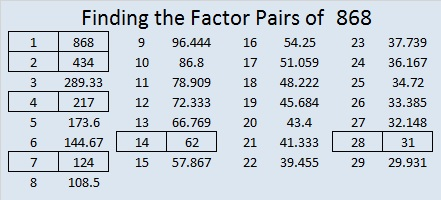# Palindrome 868 Has a Fun Square Root

Obviously, 868 is a palindrome in base 10. It has some interesting representations in some other bases, too:

• 868 is 4004 in BASE 6, because 4(6³) + 0(6²) + 0(6¹) + 4(6º) = 868
• SS in BASE 30 (S is 28 base 10), because 28(30) + 28(1) = 28(31) = 868
• S0 in BASE 31, because 28(31) = 868

From Stetson.edu I learned that all the digits from 1 to 9 make up the first nine decimal places of √868, so I decided to make a gif showing that fun fact:make science GIFs like this at MakeaGif
• 868 is a composite number.
• Prime factorization: 868 = 2 × 2 × 7 × 31, which can be written 868 = 2² × 7 × 31
• The exponents in the prime factorization are 2, 1, and 1. Adding one to each and multiplying we get (2 + 1)(1 + 1)(1 + 1) = 3 × 2 × 2 = 12. Therefore 868 has exactly 12 factors.
• Factors of 868: 1, 2, 4, 7, 14, 28, 31, 62, 124, 217, 434, 868
• Factor pairs: 868 = 1 × 868, 2 × 434, 4 × 217, 7 × 124, 14 × 62, or 28 × 31,
• Taking the factor pair with the largest square number factor, we get √868 = (√4)(√217) = 2√217 ≈ 29.461839725